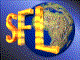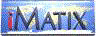| iMatix home page | << | < | > | >>SFLVersion 2.11

### conv_time_pict

```#include "sflconv.h"
char *
conv_time_pict (
long time,
const char *picture)
```

#### Synopsis

Converts a time to a string using a picture. The picture is composed of any combination of these formats:
 h hour, 0-23 hh hour, 00- 23 m minute, 0-59 mm minute, 00-59 s second, 0-59 ss second, 00-59 c centisecond, 0- 99 cc centisecond, 00-99 a a/p indicator - use 12-hour clock aa am/pm indicator - use 12-hour clock A A/P indicator - use 12-hour clock AA AM/PM indicator - use 12-hour clock \x literal character x other literal character
Returns the formatted result. This is a static string, of at most 80 characters, that is overwritten by each call. If time is zero, returns an empty string. The 'h', 'm', 's', and 'c' formats output a leading space when used at the start of the picture. This is to improve the alignment of a column of times. If the previous character was a digit, these formats also output a space in place of the leading zero.

#### Source Code - (sflcvtp.c)

```{
static char
formatted [FORMAT_MAX + 1];     /*  Formatted return string          */
int
hour,                           /*  Hour component of time           */
minute,                         /*  Minute component of time         */
second,                         /*  Second component of time         */
centi,                          /*  1/100 sec component of time      */
cursize;                        /*  Size of current component        */
char
*dest,                           /*  Store formatted data here        */
ch,                             /*  Next character in picture        */
lastch = '0';                   /*  Last character we output         */
Bool
pm;                             /*  TRUE when hour >= 12             */

conv_reason = 0;                    /*  No conversion errors so far      */

/*  Zero time is returned as empty string                                */
if (time == 0)
{
strclr (formatted);
return (formatted);
}

hour    = GET_HOUR   (time);
minute  = GET_MINUTE (time);
second  = GET_SECOND (time);
centi   = GET_CENTI  (time);

/*  If am/pm component specified, use 12-hour clock                      */
if (hour >= 12)
{
pm = TRUE;
if (strpbrk (picture, "aA") && hour > 12)
hour -= 12;
}
else
pm = FALSE;

ASSERT (hour   >= 0 && hour   < 24);
ASSERT (minute >= 0 && minute < 60);
ASSERT (second >= 0 && second < 60);

/*  Scan through picture, converting each component                      */
dest = formatted;
*dest = 0;                          /*  string is empty                  */
while (*picture)
{
/*  Get character and count number of occurences                     */
ch = *picture++;
for (cursize = 1; *picture == ch; cursize++)
picture++;

switch (ch)
{
/*  h         hour,  0-23                                        */
/*  hh        hour, 00-23                                        */
case 'h':
if (cursize == 1)
sprintf (dest, (isdigit (lastch)? "%2d": "%d"), hour);
else
if (cursize == 2)
sprintf (dest, "%02d", hour);
break;

/*  m         minute,  0-59                                      */
/*  mm        minute, 00-59                                      */
case 'm':
if (cursize == 1)
sprintf (dest, (isdigit (lastch)? "%2d": "%d"), minute);
else
if (cursize == 2)
sprintf (dest, "%02d", minute);
break;

/*  s         second,  0-59                                      */
/*  ss        second, 00-59                                      */
case 's':
if (cursize == 1)
sprintf (dest, (isdigit (lastch)? "%2d": "%d"), second);
else
if (cursize == 2)
sprintf (dest, "%02d", second);
break;

/*  c         centisecond,  0-99                                 */
/*  cc        centisecond, 00-99                                 */
case 'c':
if (cursize == 1)
sprintf (dest, (isdigit (lastch)? "%2d": "%d"), centi);
else
if (cursize == 2)
sprintf (dest, "%02d", centi);
break;

/*  a         a/p indicator                                      */
/*  aa        am/pm indicator                                    */
case 'a':
strncat (dest, (pm? "pm": "am"), cursize);
dest [cursize] = 0;
break;

/*  A         A/P indicator                                      */
/*  AA        AM/PM indicator                                    */
case 'A':
strncat (dest, (pm? "PM": "AM"), cursize);
dest [cursize] = 0;
break;

/*  \x        literal character x                                */
case '\\':
ch = *picture++;
}
if (*dest)                      /*  If something was output,         */
 | << | < | > | >>Copyright © 1996-2000 iMatix Corporation